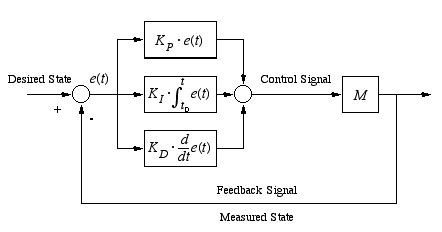Published: September 25, 2014, Edited by: Mads Hobye

# PID Controller for ArduinoMotor control can be tricky. Striking the right balance between just enough energy needed to move the motor and not too much requires a more ellaborate feedback loop than "if xx then move up" etc.. This is where a PID controller comes in:

A proportional-integral-derivative controller (PID controller) is a control loop feedback mechanism (controller) widely used in industrial control systems. A PID controller calculates an error value as the difference between a measured process variable and a desired setpoint. The controller attempts to minimize the error by adjusting the process through use of a manipulated variable.

The PID controller algorithm involves three separate constant parameters, and is accordingly sometimes called three-term control: the proportional, the integral and derivative values, denoted P, I, and D. Simply put, these values can be interpreted in terms of time: P depends on the present error.
I on the accumulation of past errors
D is a prediction of future errors, based on current rate of change.
 The weighted sum of these three actions is used to adjust the process via a control element such as the position of a control valve, a damper, or the power supplied to a heating element. Wikipedia

We currently had this problem when trying to control a moving head for an interactive robot platform. This consisted of a geared motor + motor controller and a potmeter wired directly to the moving axcis of the head.

``````#ifndef __SERVOPID
#define __SERVOPID
#include <arduino.h>
#define h1 3 // H-Bridge input 1
#define h2 5// H-Bridge input 2

class PIDServo
{
private:

float P_GAIN;
float I_GAIN;
float D_GAIN;

float I;
float D;
float I_LIM;
float E0;

float GAIN;
float error;

public:
boolean stop;
int sensorValue;
float SETPOINT;
int gotoPoint;

void begin()
{
// Start values
P_GAIN = 1; // Properpotional gain fejlen som bliver sendt til motoren
I_GAIN = 0.05; // lack gaing - hvis den ikke kan komme igang
D_GAIN = 0.5; // leader jerke
GAIN=1.5; // multiplication factor
I_LIM = 200;
stop = false;
gotoPoint = 312;
I = 0;
D=0;

E0=0;
SETPOINT = 312;
error=0;

//    TCCR2A = (TCCR2A & 0b11111100) | 0b00000011;

byte mode=0x01;
TCCR2B = TCCR2B & 0b11111000 | mode;

digitalWrite(h2,LOW);
analogWrite(h1,0);

pinMode(h2,OUTPUT);

}

void update()
{
SETPOINT = gotoPoint;
//SETPOINT = SETPOINT * 0.2 + (float)(gotoPoint) * 0.8f;

error=((float)sensorValue-SETPOINT)*GAIN;
D=D*0.0+(error-E0)*1.0;
E0=error;
I+=error*I_GAIN;
if(I>I_LIM)
I=I_LIM;
if(I<-I_LIM)
I=-I_LIM;
float r=error*P_GAIN+I+D*D_GAIN;

if(r>150)
r=150;
if(r<-150)
r=-150;

if(stop)
{
r = 0;
}

if(r>0)
{
//      analogWrite(h2,r);
//      analogWrite(h1,0);

//      analogWrite(h2,r);
digitalWrite(h2,HIGH);
analogWrite(h1,255-r);

}
else
{
//      analogWrite(h2,0);
digitalWrite(h2,LOW);
analogWrite(h1,-r);
}

}

void setMoving(int f)
{

gotoPoint = constrain(f,200,800);
}

};

#endif
``````

This control as motor which uses digitalwrite for direction,analogWrite for speed and analogRead to get the current position. You can change this accordingly to the needs of your application.

Add .h file to your project with the code above and initialize it in the following way:

``````#include "servopid.h"
PIDServo servo;
unsigned long updateLastTime = 0;

void setup()
{

servo.begin();
}

void loop()
{
if(millis() - updateLastTime > 20)
{
updateLastTime= millis();
servo.update();
}
servo.setMoving(512); // center point
}
``````

For the PID controller to have a proper response - quite a lot of tweaking needs to be done. Specifically, the following parameter needs to be tweaked:

``````P_GAIN = 1; // Properpotional gain.
I_GAIN = 0.05; // if it cannot start